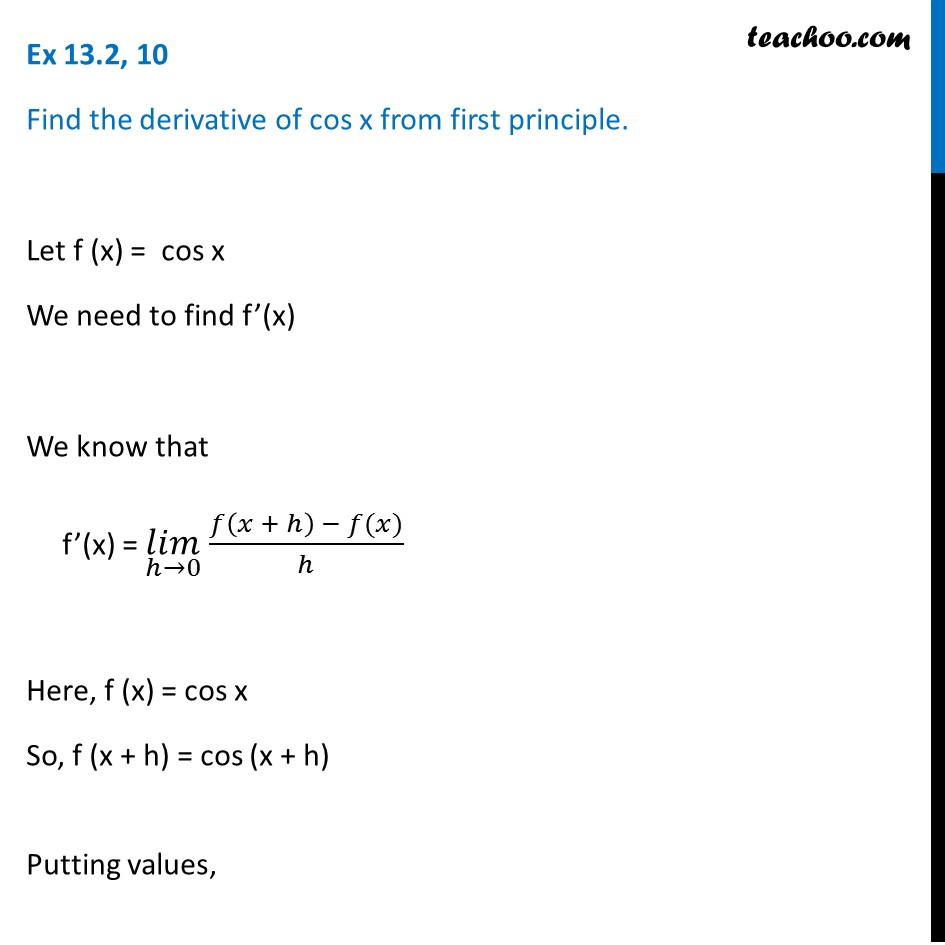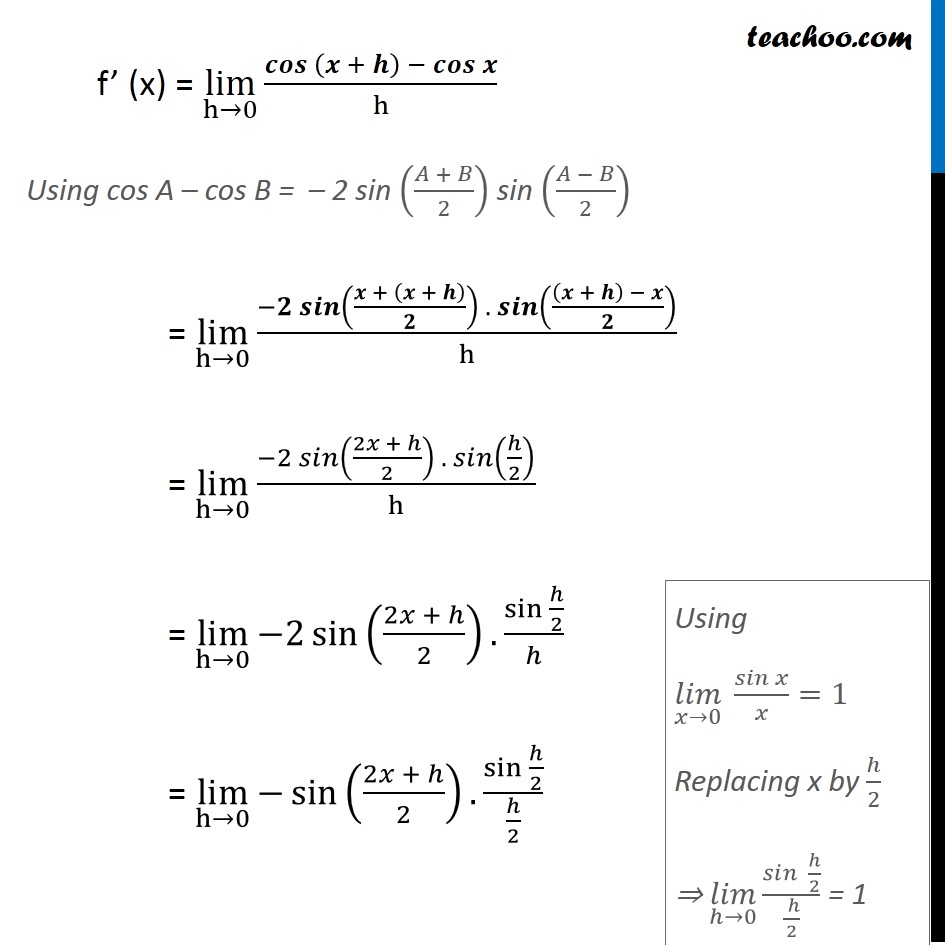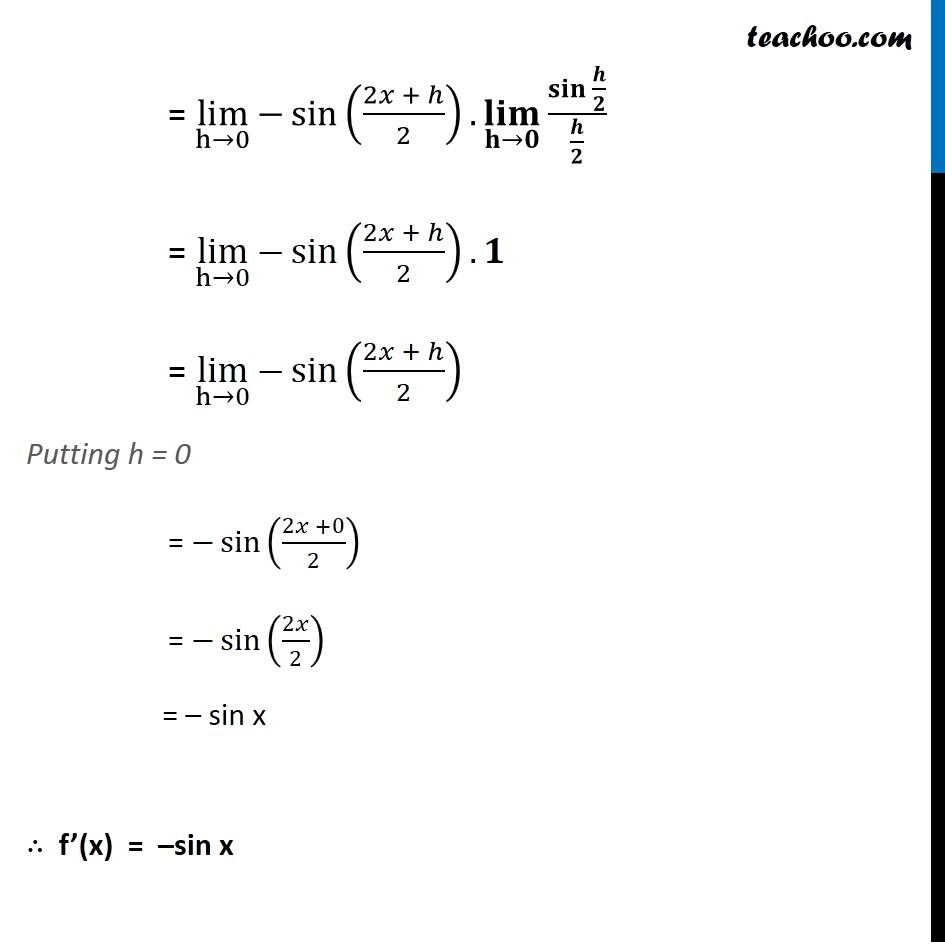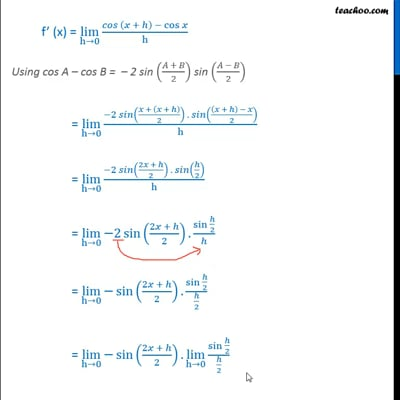Derivatives by formula - sin & cos

Chapter 13 Class 11 Limits and Derivatives
Concept wiseThis video is only available for Teachoo black users

Solve all your doubts with Teachoo Black (new monthly pack available now!)

### Transcript

Ex 13.2, 10 Find the derivative of cos x from first principle. Let f (x) = cos x We need to find f’(x) We know that f’(x) = (𝑙𝑖𝑚)┬(ℎ→0) 𝑓⁡〖(𝑥 + ℎ) − 𝑓(𝑥)〗/ℎ Here, f (x) = cos x So, f (x + h) = cos (x + h) Putting values, f’ (x) = lim┬(h→0)⁡〖(𝒄𝒐𝒔 (𝒙 + 𝒉) −〖 𝒄𝒐𝒔〗⁡𝒙)/h〗 Using cos A – cos B = – 2 sin ((𝐴 + 𝐵)/2) sin ((𝐴 − 𝐵)/2) = lim┬(h→0)⁡〖(−𝟐 𝒔𝒊𝒏((𝒙 + (𝒙 + 𝒉))/𝟐) . 𝒔𝒊𝒏(((𝒙 + 𝒉) − 𝒙)/𝟐))/h〗 = lim┬(h→0)⁡〖(−2 𝑠𝑖𝑛((2𝑥 + ℎ)/2) . 𝑠𝑖𝑛(ℎ/2))/h〗 = lim┬(h→0)⁡〖−2 sin⁡((2𝑥 + ℎ)/2).〖sin 〗⁡〖ℎ/2〗/ℎ〗 = lim┬(h→0)⁡〖−sin⁡((2𝑥 + ℎ)/2).〖sin 〗⁡〖ℎ/2〗/(ℎ/2)〗 Using (𝑙𝑖𝑚)┬(𝑥→0)⁡〖 𝑠𝑖𝑛⁡𝑥/𝑥〗=1 Replacing x by ℎ/2 ⇒ (𝑙𝑖𝑚)┬(ℎ→0) 𝑠𝑖𝑛⁡〖 ℎ/2〗/(( ℎ)/2) = 1 = lim┬(h→0)⁡〖−sin⁡((2𝑥 + ℎ)/2).(𝐥𝐢𝐦)┬(𝐡→𝟎) 〖𝐬𝐢𝐧 〗⁡〖𝒉/𝟐〗/(𝒉/𝟐)〗 = lim┬(h→0)⁡〖−sin⁡((2𝑥 + ℎ)/2).𝟏〗 = lim┬(h→0)⁡〖−sin⁡((2𝑥 + ℎ)/2) 〗 Putting h = 0 = −sin⁡((2𝑥 +0)/2) = −sin⁡(2𝑥/2) = – sin x ∴ f’(x) = –sin x